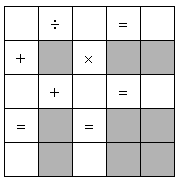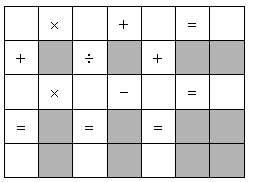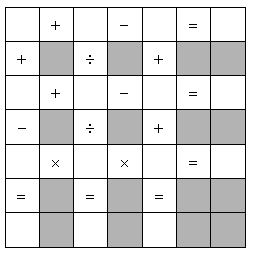Number Puzzles

Each empty white square in the grids below contains one of the numbers 1, 2, 3, . . . up to n, where n is the number of empty squares. Each of the horizontal and vertical equations should be true. Each number will be used exactly once.

Each puzzle below has a unique solution.